Back to Table of Contents [TOP] 6. Chemical Kinetics - (i) - Introduction and Experimental techniques.   The Rates of Chemical reactions  (0-1) Introduction (0-2) Experimental techniques            (0-2-1) Non Disruptive techniques            (0-2-2) Disruptive techniques: Chemical Analysis Method            (0-2-3) Special cases: fast reactions [ TOP ] (6-1) The Rates of Chemical reactions - Introduction  In general, the rate of reaction (instantaneous) for:is given by: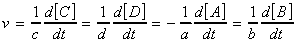This means that with molar concentrations, reaction rates are reported in mol L-1 s-1 . This quantity represents the slope of the tangent drawn to the curve showing the variation of concentration with time. For negative slopes (reactants), the sign is changed when reporting the rate, so all reaction rates are positive. This is illustarted in Fig. 0.Fig. 0: The definition of (instantaneous) rate as the slope of the tangent drawn to the curve showing the variation of concentration with time. For negative slopes, the sign is changed when reporting the rate, so all reaction rates are positive. This rate of reaction is given by the rate law, an equation that expresses the rate of the reaction as a function of all the species present in the overall chemical reaction at some time, (including catalysts, or, inhibitors) i.e.: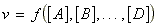It is important to note that: The rate law bears no simple relationship to the stoichiometric equation. The rate law may depend on species that do not appear in the overall reaction (e.g. catalysts). The rate law may not involve all of the species that appear in the reaction. For many reactions, the rate law assumes the form:where [A], [B], etc. are the species (reactants or products) involved in the reaction. This equation allows us to define two new terms: (i) The rate constant, k - a constant that is independent of the concentrations but dependent of temperature, (ii) The order of the reaction, which is given by 'a+ b + ' .  (a) Note that if for example, v = k[A][B], then the rate law would be first-order with respect to A, but second order overall.  (b) Note also that the order need not be an integer (in fact, many gas phase reactions involve fractional order. Other reaction (heterogeneous reactions only) may have zero-order, (i.e. v = k) i.e. their rate is independent of the concentrations. An example of a zero-order reaction is the decomposition of phosphine (PH3) on hot tungsten at high pressures. Note that not all reactions have a rate law in the form of. For example, for the simple reaction: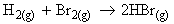the rate law is given by:An overall order for such reactions cannot be defined. (The only thing that can be said, is that the reaction is first order with respect to H2. From this discussion it becomes obvious that the study of chemical kinetics involves three main stages:   Identification of the rate law and the calculation of k from experiments; The construction of reaction mechanisms that are consistent with the rate law; The justification for the value of k and its temperature dependence. However, before we indulge into all this, let us have a look at some of the experimental techniques employed for studying reaction kinetics. [ TOP ] (6-2) Experimental techniques in chemical kinetics  In order to determine the rate of a particular reaction, it is necessary to follow either:  (a) The rate of decrease in concentration of a reactant, or (b) The rate of increase in concentration of one of the products, by choosing an appropriate experimental method.  The basic requirements are: A good thermostat as rates change exponentially with temperature An accurate timing device A method of determining the concentration of reactant or product. This is done through a measurement of a rapidly determinable physical property of the reaction mixture. [ TOP ] (6-2-1) Non Disruptive techniques:  Non-disruptive techniques (real time analysis) avoids changing the reaction mixture during the experimental measurement and thereby changing the reaction conditions (e.g. chemical analysis requiring the withdrawal of a sample has this limitation.)  Spectrophotometric Methods This usually involves selecting a suitable peak in the absorption spectrum of either a reactant or a product (the latter, if side-reactions involving a secondary reaction with the reactant are likely) and measuring absorbance as a function of time.  Beer-Lanbert law states that when a monochromatic radiation passes through a medium of thickness l, the intensity of the transmitted light, Itrans, is related to the intensity of the incident light I0 by: The absorbance A is defined as: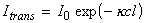where k is known as the molar Napierian absorption coefficient, and c is the concentration of the absorbing medium. Thus taking logs we get: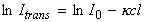i.e.: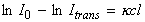i.e.:More commonly we make use of the absorbance, A, defined as: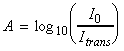i.e.:where e (=k / ln10) is known as the extinction coefficient. If we now consider a unimolecular reaction R -> P. If A is the absorbance due to product P when the reaction has gone to completion, and At is the absorbance at ant time t, then the difference (A-At) is proportional to the concentration of the reactant at any time t, while (A-A0) is proportional to the initial concentration.  Other 'non-disruptive' methods: (A) Gas Reactions from Change in Total Pressure The total pressure at any time during a gas-phase reaction will be the sum of the partial pressures of all the gaseous reactant and product molecules. Thus if the stoichiometry of the reaction is known, this can be used to enable the rate of decrease of reactant with time to be determined as a function of the measured total pressure. Side-reactions also cause a problem with this technique and the pressure must also be measured at constant volume. This method is illustrated in Worked Example 4.11.7. (B) Electrical Conductivity Method Consider, for example, the reaction:The conductivity of hydroxyl (and hydrogen) ions greatly exceeds that of other ions such as ethanoate ions. Therefore a measurement of the conductivity is an appropriate method for following the rate of loss of hydroxyl ions in the above process.  (C) Optical Rotation Method Suitable for reactions where the optical activity of the products are different from those of the reactants, e.g.:In such cases, a measurement of the optical rotation will be related to the change in concentration. (D) Refractive Index Method A method which is useful for liquid-phase reactions where the refractive index of raection mixture changes in the course of the reaction due to increase in the products/decrease in the reactants. (E) Dilatometry Method This follows the reaction by measuring the volume change during a liquid-phase reaction. (F) Gas Evolution Method If there is one gaseous product in a reaction, the gas evolved can be collected and its volume measured as a function of time. For example, the decomposition of benzenediazonium chloride proceeds via: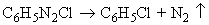and the rate constant can be determined from a measurement of the volume of nitrogen evolved during the reaction. [ TOP ] (6-2-2) Disruptive techniques: Chemical Analysis Method In chemical analysis method, a sample is taken from the reaction mixture and analyzed, by for example, titration to estimate the acid liberated during the hydrolysis of an ester, of running a gas chromatograph .  If chemical analysis is to be employed precautions must be taken to ensure that no further reaction takes place between sampling and analysis (quenching). This is usually achieved by: liberal dilution of the reaction mixture, and/or  a lowering of the temperature immediately after sampling. rapidly neutralizing an acid/base reactant. Another disadvantage of this technique is that normally one reaction mixture is needed for every sample (i.e. every reading) whereas this is not the case for all the previous techniques. This is because when a sample is removed, the reaction conditions are changed. This disadvantage may be treated by taking very small samples. (The use of gas chromatography and other similar analytical tools means that very small samples can be withdrawn at set times without significant changes in reactant concentration occurring.) [ TOP ] (6-2-3) Special cases: fast reactions The methods described above, especially disruptive techniques, are suitable only for reactions that are slow enough for there to be little reaction during the time it takes to quench the mixture or to make the measurement. Nowadays, the 'in vogue' research is to study fast reactions, i.e. reactions which are complete in less than about 1 s (and often very much less). The present thrust of chemical kinetics is to ever shorter timescales, and with special laser techniques it is now possible to observe processes occurring in a few tens of femtoseconds. Reactions that take place in times as short as one second or less need special techniques involving flow methods or stopped-flow methods. Flash photolysis and shock-wave kinetic experiments are also relatively modern techniques for studying fast reactions where spectroscopic measurements are often used for analysis of the reaction mixture. In the flow method the reactants are mixed as they flow together in a chamber (Fig. 1a). The reaction continues as the thoroughly mixed solutions flow through the outlet tube, and observation of the composition at different positions along the tube is equivalent to the observation of the reaction mixture at different times after mixing. The disadvantage of conventional flow techniques is that a large volume of reactant solution is necessary. This disadvantage is particularly important for fast reactions, because to spread the reaction over a length of tube the flow must be rapid.  The stopped-flow technique avoids this disadvantage (Fig. 1b). The suitability of the stopped-flow technique to the study of small samples means that it is appropriate for biochemical reactions, and it has been widely used to study the kinetics of enzyme action.  In flash photolysis, the gaseous or liquid sample is exposed to a brief photolytic flash of light, and then the contents of the reaction chamber are monitored. Most work is now done with lasers with flashes of about 10 ns duration, but many studies are carried out at 1 ps, and some are done on a femtosecond timescale. Either emission or absorption spectroscopy may be used to monitor the reaction, and the spectra are recorded electronically at a series of times following the flash.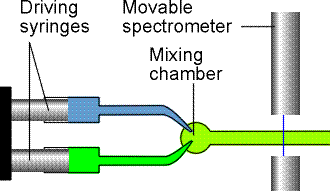Fig. 1a: The arrangement used in the flow technique for studying reaction rates. The reactants are injected into the mixing chamber at a steady rate. The location of the spectrometer corresponds to different times after initiation.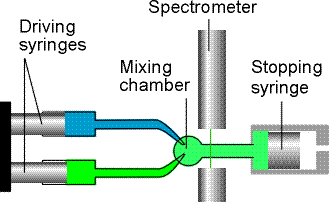Fig. 1b: In the stopped-flow technique the reagents are driven quickly into the mixing chamber by the driving syringes and then the time dependence of the concentrations is monitored.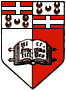CH237 - Chemical Thermodynamics and Kinetics

 BACK TO TABLE OF CONTENTS   7. Chemical Kinetics - (i) - Introduction and Experimental techniques.   The Rates of Chemical reactions  (0-1) Introduction (0-2) Experimental techniques            (0-2-1) Non Disruptive techniques            (0-2-2) Disruptive techniques: Chemical Analysis Method            (0-2-3) Special cases: fast reactions [ TOP ] (0-1) The Rates of Chemical reactions - Introduction  In general, the rate of reaction (instantaneous) for:is given by:This means that with molar concentrations, reaction rates are reported in mol L-1 s-1 . This quantity represents the slope of the tangent drawn to the curve showing the variation of concentration with time. For negative slopes (reactants), the sign is changed when reporting the rate, so all reaction rates are positive. This is illustarted in Fig. 0.Fig. 0: The definition of (instantaneous) rate as the slope of the tangent drawn to the curve showing the variation of concentration with time. For negative slopes, the sign is changed when reporting the rate, so all reaction rates are positive. This rate of reaction is given by the rate law, an equation that expresses the rate of the reaction as a function of all the species present in the overall chemical reaction at some time, (including catalysts, or, inhibitors) i.e.:It is important to note that: The rate law bears no simple relationship to the stoichiometric equation. The rate law may depend on species that do not appear in the overall reaction (e.g. catalysts). The rate law may not involve all of the species that appear in the reaction. For many reactions, the rate law assumes the form:where [A], [B], etc. are the species (reactants or products) involved in the reaction. This equation allows us to define two new terms: (i) The rate constant, k - a constant that is independent of the concentrations but dependent of temperature, (ii) The order of the reaction, which is given by 'a+ b + ' .  (a) Note that if for example, v = k[A][B], then the rate law would be first-order with respect to A, but second order overall.  (b) Note also that the order need not be an integer (in fact, many gas phase reactions involve fractional order. Other reaction (heterogeneous reactions only) may have zero-order, (i.e. v = k) i.e. their rate is independent of the concentrations. An example of a zero-order reaction is the decomposition of phosphine (PH3) on hot tungsten at high pressures. Note that not all reactions have a rate law in the form of. For example, for the simple reaction:the rate law is given by:An overall order for such reactions cannot be defined. (The only thing that can be said, is that the reaction is first order with respect to H2. From this discussion it becomes obvious that the study of chemical kinetics involves three main stages:   Identification of the rate law and the calculation of k from experiments; The construction of reaction mechanisms that are consistent with the rate law; The justification for the value of k and its temperature dependence. However, before we indulge into all this, let us have a look at some of the experimental techniques employed for studying reaction kinetics. [ TOP ] (0-2) Experimental techniques in chemical kinetics  In order to determine the rate of a particular reaction, it is necessary to follow either:  (a) The rate of decrease in concentration of a reactant, or (b) The rate of increase in concentration of one of the products, by choosing an appropriate experimental method.  The basic requirements are: A good thermostat as rates change exponentially with temperature An accurate timing device A method of determining the concentration of reactant or product. This is done through a measurement of a rapidly determinable physical property of the reaction mixture. [ TOP ] (0-2-1) Non Disruptive techniques:  Non-disruptive techniques (real time analysis) avoids changing the reaction mixture during the experimental measurement and thereby changing the reaction conditions (e.g. chemical analysis requiring the withdrawal of a sample has this limitation.)  Spectrophotometric Methods This usually involves selecting a suitable peak in the absorption spectrum of either a reactant or a product (the latter, if side-reactions involving a secondary reaction with the reactant are likely) and measuring absorbance as a function of time.  Beer-Lanbert law states that when a monochromatic radiation passes through a medium of thickness l, the intensity of the transmitted light, Itrans, is related to the intensity of the incident light I0 by: The absorbance A is defined as:where k is known as the molar Napierian absorption coefficient, and c is the concentration of the absorbing medium. Thus taking logs we get:i.e.:i.e.:More commonly we make use of the absorbance, A, defined as:i.e.:where e (=k / ln10) is known as the extinction coefficient. If we now consider a unimolecular reaction R -> P. If A is the absorbance due to product P when the reaction has gone to completion, and At is the absorbance at ant time t, then the difference (A-At) is proportional to the concentration of the reactant at any time t, while (A-A0) is proportional to the initial concentration.  Other 'non-disruptive' methods: (A) Gas Reactions from Change in Total Pressure The total pressure at any time during a gas-phase reaction will be the sum of the partial pressures of all the gaseous reactant and product molecules. Thus if the stoichiometry of the reaction is known, this can be used to enable the rate of decrease of reactant with time to be determined as a function of the measured total pressure. Side-reactions also cause a problem with this technique and the pressure must also be measured at constant volume. This method is illustrated in Worked Example 4.11.7. (B) Electrical Conductivity Method Consider, for example, the reaction:The conductivity of hydroxyl (and hydrogen) ions greatly exceeds that of other ions such as ethanoate ions. Therefore a measurement of the conductivity is an appropriate method for following the rate of loss of hydroxyl ions in the above process.  (C) Optical Rotation Method Suitable for reactions where the optical activity of the products are different from those of the reactants, e.g.:In such cases, a measurement of the optical rotation will be related to the change in concentration. (D) Refractive Index Method A method which is useful for liquid-phase reactions where the refractive index of raection mixture changes in the course of the reaction due to increase in the products/decrease in the reactants. (E) Dilatometry Method This follows the reaction by measuring the volume change during a liquid-phase reaction. (F) Gas Evolution Method If there is one gaseous product in a reaction, the gas evolved can be collected and its volume measured as a function of time. For example, the decomposition of benzenediazonium chloride proceeds via:and the rate constant can be determined from a measurement of the volume of nitrogen evolved during the reaction. [ TOP ] (0-2-2) Disruptive techniques: Chemical Analysis Method In chemical analysis method, a sample is taken from the reaction mixture and analyzed, by for example, titration to estimate the acid liberated during the hydrolysis of an ester, of running a gas chromatograph .  If chemical analysis is to be employed precautions must be taken to ensure that no further reaction takes place between sampling and analysis (quenching). This is usually achieved by: liberal dilution of the reaction mixture, and/or  a lowering of the temperature immediately after sampling. rapidly neutralizing an acid/base reactant. Another disadvantage of this technique is that normally one reaction mixture is needed for every sample (i.e. every reading) whereas this is not the case for all the previous techniques. This is because when a sample is removed, the reaction conditions are changed. This disadvantage may be treated by taking very small samples. (The use of gas chromatography and other similar analytical tools means that very small samples can be withdrawn at set times without significant changes in reactant concentration occurring.) [ TOP ] (0-2-3) Special cases: fast reactions The methods described above, especially disruptive techniques, are suitable only for reactions that are slow enough for there to be little reaction during the time it takes to quench the mixture or to make the measurement. Nowadays, the 'in vogue' research is to study fast reactions, i.e. reactions which are complete in less than about 1 s (and often very much less). The present thrust of chemical kinetics is to ever shorter timescales, and with special laser techniques it is now possible to observe processes occurring in a few tens of femtoseconds. Reactions that take place in times as short as one second or less need special techniques involving flow methods or stopped-flow methods. Flash photolysis and shock-wave kinetic experiments are also relatively modern techniques for studying fast reactions where spectroscopic measurements are often used for analysis of the reaction mixture. In the flow method the reactants are mixed as they flow together in a chamber (Fig. 1a). The reaction continues as the thoroughly mixed solutions flow through the outlet tube, and observation of the composition at different positions along the tube is equivalent to the observation of the reaction mixture at different times after mixing. The disadvantage of conventional flow techniques is that a large volume of reactant solution is necessary. This disadvantage is particularly important for fast reactions, because to spread the reaction over a length of tube the flow must be rapid.  The stopped-flow technique avoids this disadvantage (Fig. 1b). The suitability of the stopped-flow technique to the study of small samples means that it is appropriate for biochemical reactions, and it has been widely used to study the kinetics of enzyme action.  In flash photolysis, the gaseous or liquid sample is exposed to a brief photolytic flash of light, and then the contents of the reaction chamber are monitored. Most work is now done with lasers with flashes of about 10 ns duration, but many studies are carried out at 1 ps, and some are done on a femtosecond timescale. Either emission or absorption spectroscopy may be used to monitor the reaction, and the spectra are recorded electronically at a series of times following the flash.Fig. 1a: The arrangement used in the flow technique for studying reaction rates. The reactants are injected into the mixing chamber at a steady rate. The location of the spectrometer corresponds to different times after initiation.Fig. 1b: In the stopped-flow technique the reagents are driven quickly into the mixing chamber by the driving syringes and then the time dependence of the concentrations is monitored.

[ UNIVERSITY OF MALTA | FACULTY OF SCIENCE | DEPARTMENT OF CHEMISTRY ]

 E-mail me at jgri1@um.edu.mt http://staff.um.edu.mt/jgri1